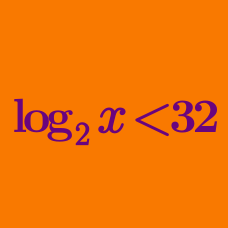Algebra

# Logarithmic Inequalities - Multiple Terms

If the range of $$x$$ that satisfies ${5}^{\log_{9}x} \cdot {x}^{\log_{9}{5}}-3({5}^{\log_{9}x}+{x}^{\log_{9}{5}})+5 < 0$ is $$\alpha < x < \beta,$$ what is the value of $$\alpha+\beta?$$

What is the range of $$x$$ that satisfies the logarithmic inequality $(\log_{4}x)^2-5\log_{4}x+6 \leq 0 ?$

If the range of $$x$$ that satisfies the logarithmic inequality $(\log_{5}x)^2-\log_{5}125x^{2} \leq 0$ is $$\alpha \leq x \leq \beta,$$ what is the value of $$\alpha\beta?$$

If the logarithmic inequality $(\log_{4}x)^2 \geq \log_{4}ax^2$ is true for all positive numbers $$x,$$ what is the range of $$a ?$$

What is the number of integers $$x$$ that satisfy $\log_{\frac{1}{2}} \frac{4}{x} \cdot \log_{\frac{1}{2}} \frac{x}{8} \geq -2 ?$

×

Problem Loading...

Note Loading...

Set Loading...